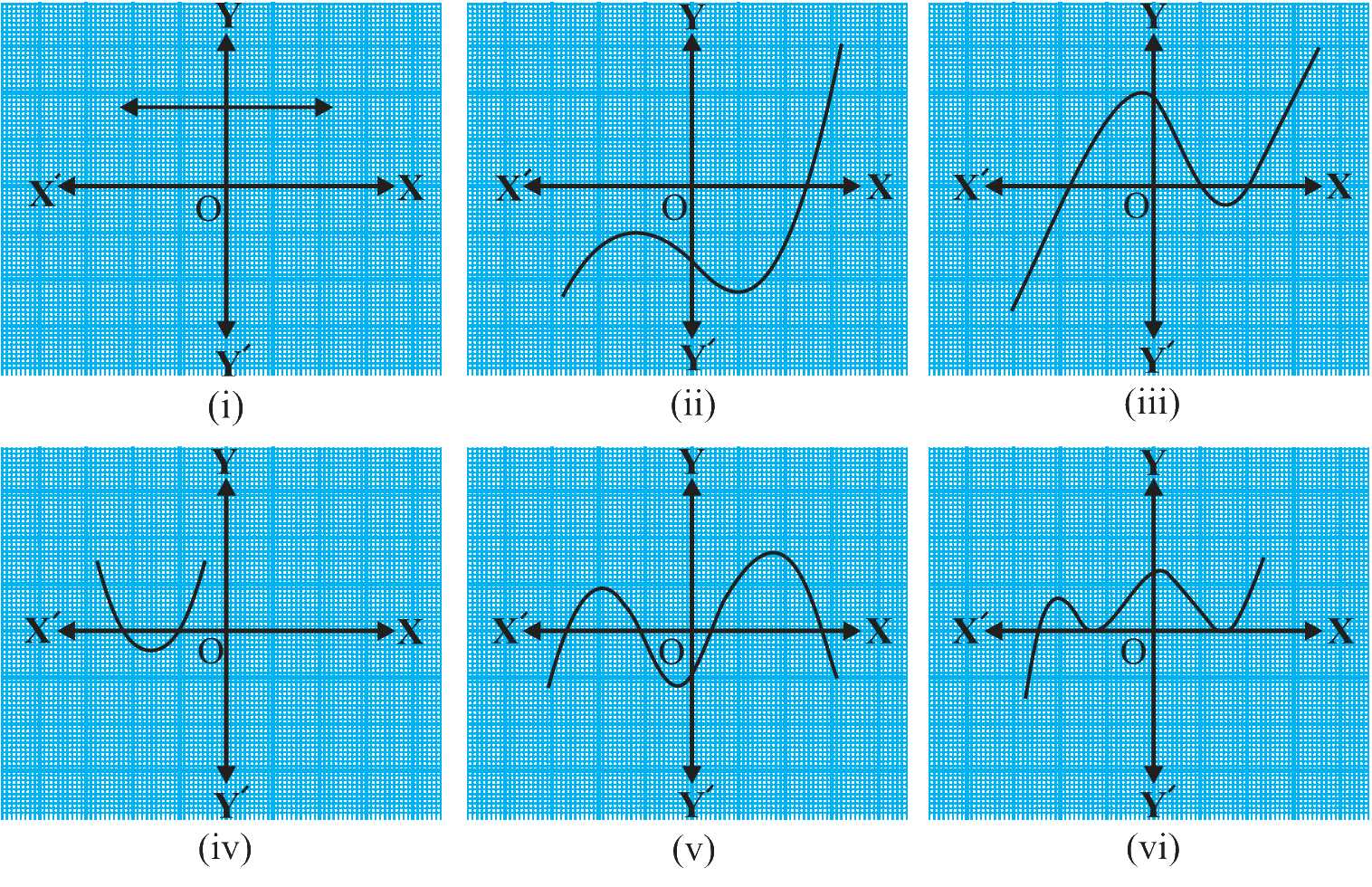# Ex.2.1 Q1 Polynomials Solution - NCERT Maths Class 10

Go back to  'Ex.2.1'

## Question

The graphs of $$y{ }={ }p\left( x \right)$$ are given in the figure below, for some polynomials $$p\left( x \right)$$. Find the number of zeroes of $$p\left( x \right)$$ in each case.Video Solution
Polynomials
Ex 2.1 | Question 1

## Text Solution

What is known?

The graphs of $$y{ }={ }p\left( x \right)$$ are given in the above figure for some polynomials $$p\left( x \right)$$.

What is unknown?

The number of zeroes of $$p\left( x \right)$$ in each case.

Reasoning:

You can reach the solution easily by understanding the statement of the question. As The graphs of $$y{ }={ }p\left( x \right)$$ are given and you have to find the number of zeroes of $$p\left( x \right)$$ in each case.

To find this look at the graphs and visually find how many points it cuts the $$x$$-axis. The number of points the graph cuts or touches the $$x$$-axis. The number of points it cut or touches the $$x$$-axis are the zeroes of the polynomial $$p\left( x \right)$$.

Steps:

(i) The number of zeroes is $$0$$ as the graph doesn’t cuts the $$x$$-axis at any point.

(ii) The number of zeroes is $$1$$ as the graph cuts the $$x$$-axis at only one point.

(iii) The number of zeroes is $$3$$ as the graph cuts the $$x$$-axis at $$3$$ points.

(iv) The number of zeroes is $$2$$ as the graph cuts the $$x$$-axis at $$2$$ points.

(v) The number of zeroes is $$4$$ as the graph cuts the $$x$$-axis at $$4$$ points.

(vi) The number of zeroes is $$3$$ as the graph cuts the $$x$$-axis at  $$3$$ points.

Learn from the best math teachers and top your exams

• Live one on one classroom and doubt clearing
• Practice worksheets in and after class for conceptual clarity
• Personalized curriculum to keep up with school